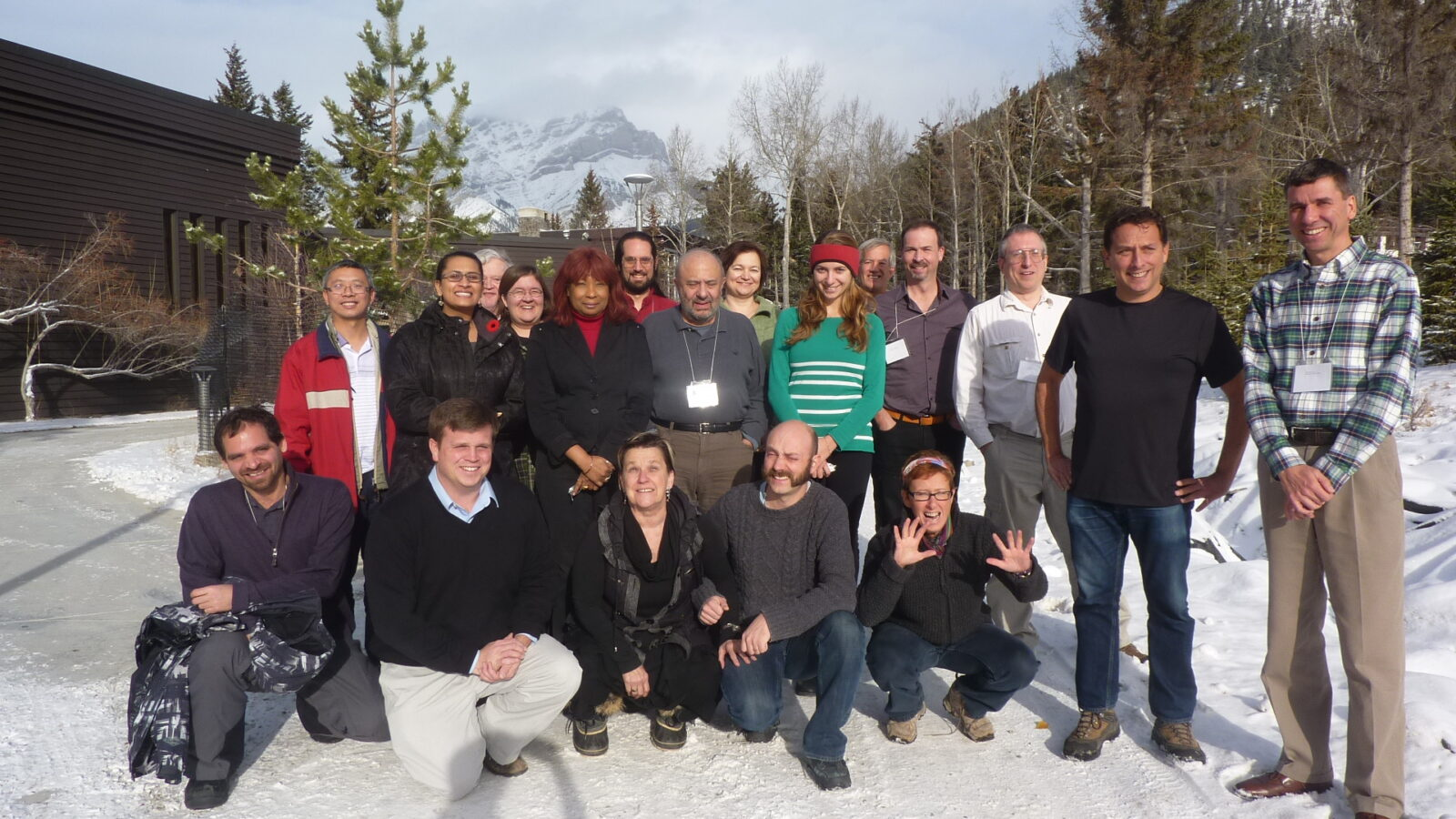Select Page

### Unsolved K-12

Only a fraction of unsolved problems are suitable for the school classroom, however there still are a huge number to choose from. The purpose of this conference was to gather mathematicians and educators together to select one unsolved problem for each grade K-12. Here is a pdf summarizing the winning unsolved problems. Here are the criteria used to make our decisions:

• The problem is curricular. For example, Goldbach’s Conjecture (all even prime numbers more than two are the sum of two prime numbers) seems good for grade six students learning about prime and composite numbers, but it emphasizes addition too much for the grade six curriculum – hence Goldbach’s Conjecture should not be selected.
• The problem is fun for the students. For example, the Gauss Lattice Point Problem (how many lattice points fit in a circle was curricular based for high school students working with conic sections, but failed to inspire many high-school students. However, the same problem worked better in grade three where the curriculum connection is area measurement.
• The problem does not confuse students. For example, exploring Multiplicative Persistence is curricular based and fun for grade 4 students learning multiplication, but a few students subsequently became confused about normal multiplication rules – hence Multiplicative Persistence needed to be discarded or explained using a distinctive operator.
• The problem is easy & cheap to implement by the teacher. For example, dice are common manipulatives in elementary schools, hence the use of dice in a grade five unsolved problem should be both easy & cheap.
• The thirteen problems are as varied as possible. Some should be easy to explain on the radio, some should be games, some should be at the intersection of art and math, etc.
• The problems should all hold stories – real or fictitious. The tragic life of Issai Schur might not be told in front of grade 2 classroom, but it can still inspire parents and teachers.
• The creators of the problems are mostly white men. This needs to be rectified over time.Unsolved K-12 Introduction to the conference and the process of selecting the 13 problems – one for each grade K-12.

Kindergarten – non-final selection. This ended up being the grade 1 unsolved problem.

Kindergarten – final selection The final selection for Kindergarten is this unsolved problem posed in 1977 by Krishnamoorthy & Deo.
Grade 1 – non-final selection The selected grade 1 problem is the beetle problem in the middle top row.
Grade 2 – final selection Sum-Free Partitions by Isaii Schur, 1916
Grade 3 – non-final selection The second problem on this video (the 1967 Graceful Tree Conjecture) is the final selection. The game, Aggression, will undoubtedly be the winning grade 3 entry for an upcoming conference, Math Games K-12.
Grade 4 – final selection Collatz conjecture, 1937
Grade 5 – non-final selection The final selection is an unsolved problem posed in 2013 by Brian Conrey et al. about non-transitive dice. You’ll see this problem described in the grade 11 video below.
Grade 6 – non-final selection The twin prime conjecture by Euclid, 250 B.C.E. is the final selection. The classroom experience will be based on Polya’s paper: Heuristic Reasoning in the Theory of Numbers. You can see the twin prime conjecture described in the grade 7 video.
Grade 7 – non-final selection The Square Tiling of a Rectangle Problem by Stewart Anderson, 2013, is the final selection.

Grade 8 – non-final selection The Erdős Strauss Conjecture is the final selection. At the conference Joshua Zucker created this way of presenting the problem that got featured here in the New York Times.

Grade 9 – non-final selection The Prime Number Catacombs by William Paulsen, 2000 is the final selection.
Grade 10 – final selection Imbedded Square Problem, Otto Toeplitz, 1911
Grade 11 – non-final selection The final selection is Markov Numbers from 1897.
Grade 12 – non-final selection The final selection is Stephen Cook’s P=NP problem from 1971.
Lior Pachter of Berkeley is pursuing unsolved problems in the classroom independently. Look at his ideas here.

Here is a pdf summarizing the winning unsolved problems.